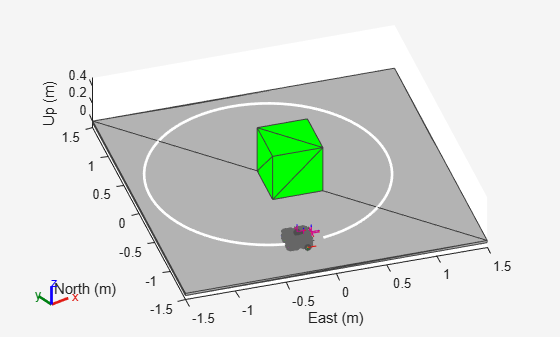# robotScenario

Generate robot simulation scenario

## Description

The `robotScenario` object generates a simulation scenario consisting of static meshes, robot platforms, and sensors in a 3-D environment.

## Creation

### Syntax

``scenario = robotScenario``
``scenario = robotScenario(Name=Value)``

### Description

````scenario = robotScenario` creates an empty robot scenario with default property values. The default inertial frames are the east-north-up (ENU) and the north-east-down (NED) frames.```

example

````scenario = robotScenario(Name=Value)` configures a `robotScenario` object with properties using one or more name-value pair arguments.```

## Properties

expand all

Simulation update rate, specified as a positive scalar in Hz. The step size of the scenario when using an `advance` object function is equal to the inverse of the update rate.

Example: `2`

Data Types: `single` | `double`

Stop time of the simulation, specified as a nonnegative scalar in seconds. A scenario stops advancing when it reaches the stop time.

Example: `60`

Data Types: `single` | `double`

Maximum number of steps stored in scenario, specified as a positive integer greater than `1`. This property determines the maximum number of frames of platform poses stored in the scenario. If the number of simulated steps exceeds the value of this property, then the scenario stores only latest steps.

Example: `60`

Data Types: `single` | `double`

Scenario origin in geodetic coordinates, specified as a three-element vector of the form ```[latitude longitude altitude]```. latitude and longitude are geodetic coordinates in degrees. altitude is the height above the WGS84 reference ellipsoid in meters.

Example: `[46.017 7.750 1673]`

Data Types: `single` | `double`

Maximum number of frames in the scenario, specified as a positive integer. The combined number of inertial frames, platforms, and sensors added to the scenario must be less than or equal to the value of this property.

Example: `15`

Data Types: `single` | `double`

Current simulation time, specified as a nonnegative scalar.

Data Types: `single` | `double`

Indicate whether the scenario is running, specified as `true` or `false`. After a scenario simulation starts, it runs until it reaches the stop time.

Data Types: `logical`

Transformation information between all the frames in the scenario, specified as a `transformTree` object. This property contains the transformation information between the inertial, platform, and sensor frames associated with the scenario.

Names of the inertial frames in the scenario, specified as a vector of strings.

Data Types: `string`

Static meshes in the scenario, specified as a 1-by-N cell array of `extendedObjectMesh` objects.

Robot platforms in the scenario, specified as an array of `robotPlatform` objects.

## Object Functions

 `addInertialFrame` Define new inertial frame in robot scenario `addMesh` Add new static mesh to robot scenario `advance` Advance robot scenario simulation by one time step `binaryOccupancyMap` Create 2-D binary occupancy map from robot scenario `restart` Reset simulation of robot scenario `setup` Prepare robot scenario for simulation `show3D` Visualize robot scenario in 3-D `updateSensors` Update sensor readings in robot scenario

## Examples

collapse all

Create a robot scenario.

`scenario = robotScenario(UpdateRate=100,StopTime=1);`

Add the ground plane and a box as meshes.

```addMesh(scenario,"Plane",Size=[3 3],Color=[0.7 0.7 0.7]); addMesh(scenario,"Box",Size=[0.5 0.5 0.5],Position=[0 0 0.25], ... Color=[0 1 0])```

Create a waypoint trajectory for the robot platform using an ENU reference frame.

```waypoint = [0 -1 0; 1 0 0; -1 1 0; 0 -1 0]; toa = linspace(0,1,length(waypoint)); traj = waypointTrajectory("Waypoints",waypoint, ... "TimeOfArrival",toa, ... "ReferenceFrame","ENU");```

Create a `rigidBodyTree` object of the TurtleBot 3 Waffle Pi robot with loadrobot.

`robotRBT = loadrobot("robotisTurtleBot3WafflePi");`

Create a robot platform with trajectory.

```platform = robotPlatform("TurtleBot",scenario, ... BaseTrajectory=traj);```

Set up platform mesh with the `rigidBodyTree` object.

`updateMesh(platform,"RigidBodyTree",Object=robotRBT)`

Create an INS sensor object and attach the sensor to the platform.

```ins = robotSensor("INS",platform,insSensor("RollAccuracy",0), ... UpdateRate=scenario.UpdateRate);```

Visualize the scenario.

```[ax,plotFrames] = show3D(scenario); axis equal hold on```

In a loop, step through the trajectory to output the position, orientation, velocity, acceleration, and angular velocity.

```count = 1; while ~isDone(traj) [Position(count,:),Orientation(count,:),Velocity(count,:), ... Acceleration(count,:),AngularVelocity(count,:)] = traj(); count = count+1; end```

Create a line plot for the trajectory. First create the plot with `plot3`, then manually modify the data source properties of the plot. This improves the performance of the plotting.

```trajPlot = plot3(nan,nan,nan,"Color",[1 1 1],"LineWidth",2); trajPlot.XDataSource = "Position(:,1)"; trajPlot.YDataSource = "Position(:,2)"; trajPlot.ZDataSource = "Position(:,3)";```

Set up the simulation. Then, iterate through the positions and show the scene each time the INS sensor updates. Advance the scene, move the robot platform, and update the sensors.

```setup(scenario) for idx = 1:count-1 % Read sensor readings. [isUpdated,insTimestamp(idx,1),sensorReadings(idx)] = read(ins); if isUpdated % Use fast update to move platform visualization frames. show3D(scenario,FastUpdate=true,Parent=ax); % Refresh all plot data and visualize. refreshdata drawnow limitrate end % Advance scenario simulation time. advance(scenario); % Update all sensors in the scene. updateSensors(scenario) end hold off```## Version History

Introduced in R2022a